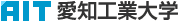日本語
 Please use this identifier to cite or link to this item: `http://hdl.handle.net/11133/632`

 Title: コンクリートの圧縮強度と引張強度の確率分布と寸法効果に関する研究(第2報) Other Titles: コンクリート ノ アッシュク キョウド ト ヒッパリ キョウド ノ カクリツ ブンプ ト スンポウコウカ ニカンスル ケンキュウ ダイ2ホウStudy on Probability Distribution and Size Effect of Compressive and Tensile Strength of Concrete (II) Authors: 小池, 狹千朗加藤, 善之助KOIKE, SachioKATO, Zennosuke Issue Date: 31-Mar-1982 Publisher: 愛知工業大学 Abstract: This study examined the probability distribution and size effect of compressive strength of three kinds of concrete cylinders and five kinds of concrete prisms and direct tensile strength of three kinds of concrete prisms by lazy tongs grips method and splitting tensile strength of three kinds of concrete cylinders, using four kinds of concrete mix proportions each having different maximum sizes of aggregates (sieve dimensions=10,15,20 and 25mm) as inclusion, and provided data to simulate occurrence of the probability distribution of concrete strength used. Direct tensile test technique was used for specimens with enlarged ends to which load was applied purely by friction using four kinds of lazy tongs grips. 1. The experimental value of strength shows a probability distribution quite close to the straight line when plotted either on Weibull probability papers or on normal ones. 2. Compressive strengths of prism and cylinder specimens show the maximum values at S=10.0cm, and decreases gradually when size specimens larger in the range where S is larger than 10cm. 3. The value of coefficient of variation CV of concrete strength increases greatly with decrease in S in the range where prism width S is 4.46-7.25 and cylinder diameters S (D) is 7.5-10.0. 4. The value of CV of prism and cylinder strength increases greatly with decrease in D/d in the range where D/d is less than 4. The size of model specimen D and size of aggregate d should be used in the range where D/d is larger than 4-5 in the tests of concrete models. URI: http://hdl.handle.net/11133/632 Appears in Collections: 17号

Files in This Item:

File Description SizeFormatDSpace Software Copyright © 2002-2010  Duraspace - Feedback